Question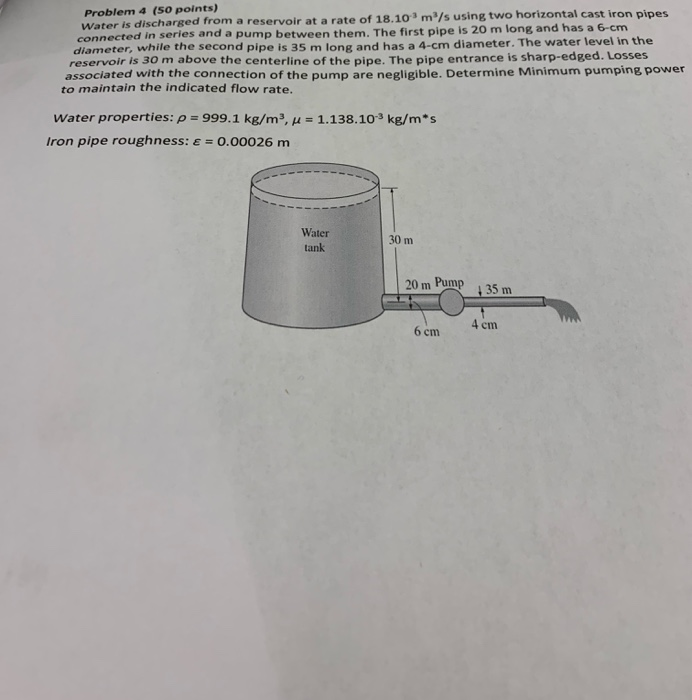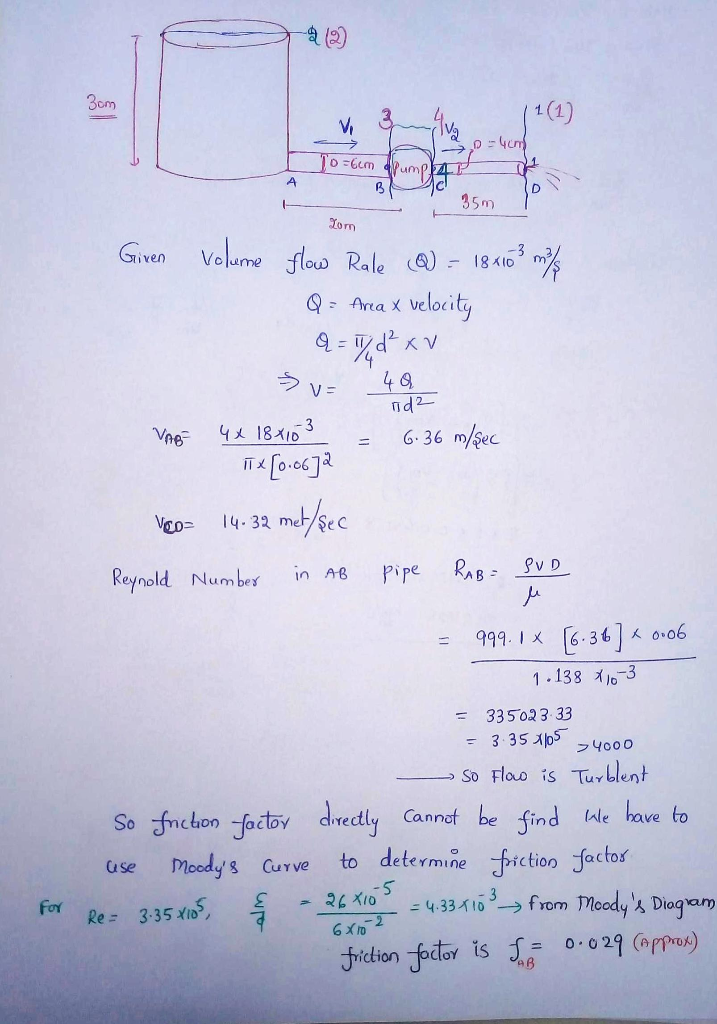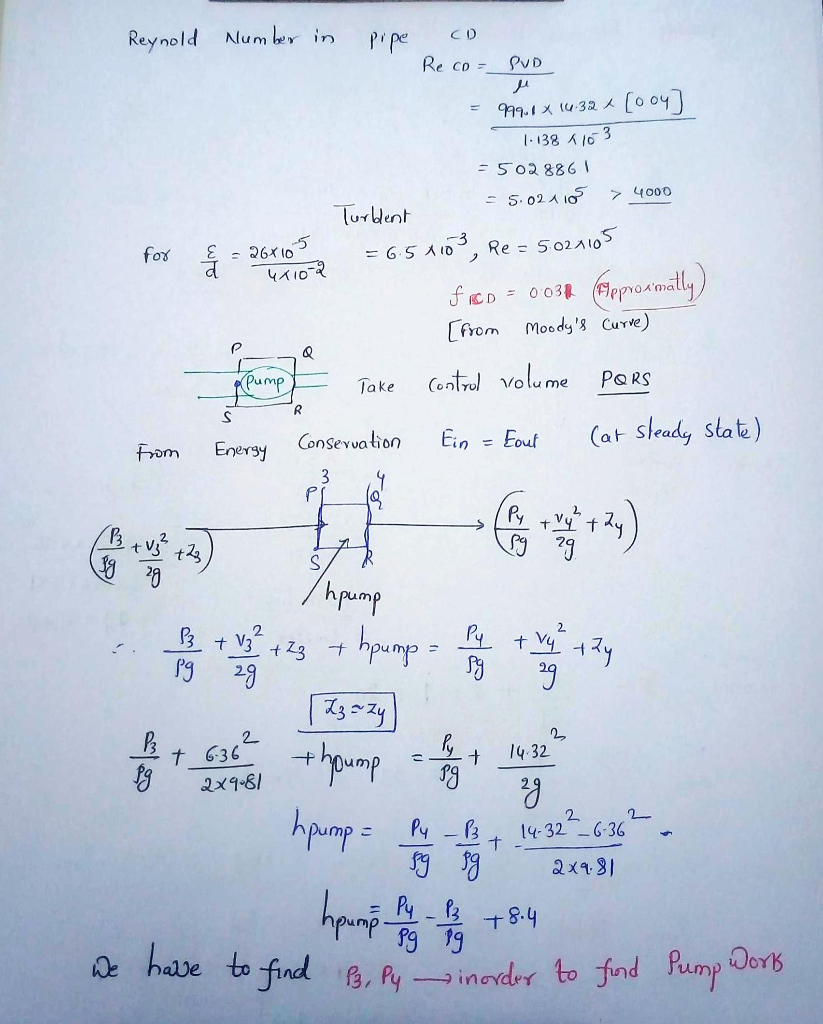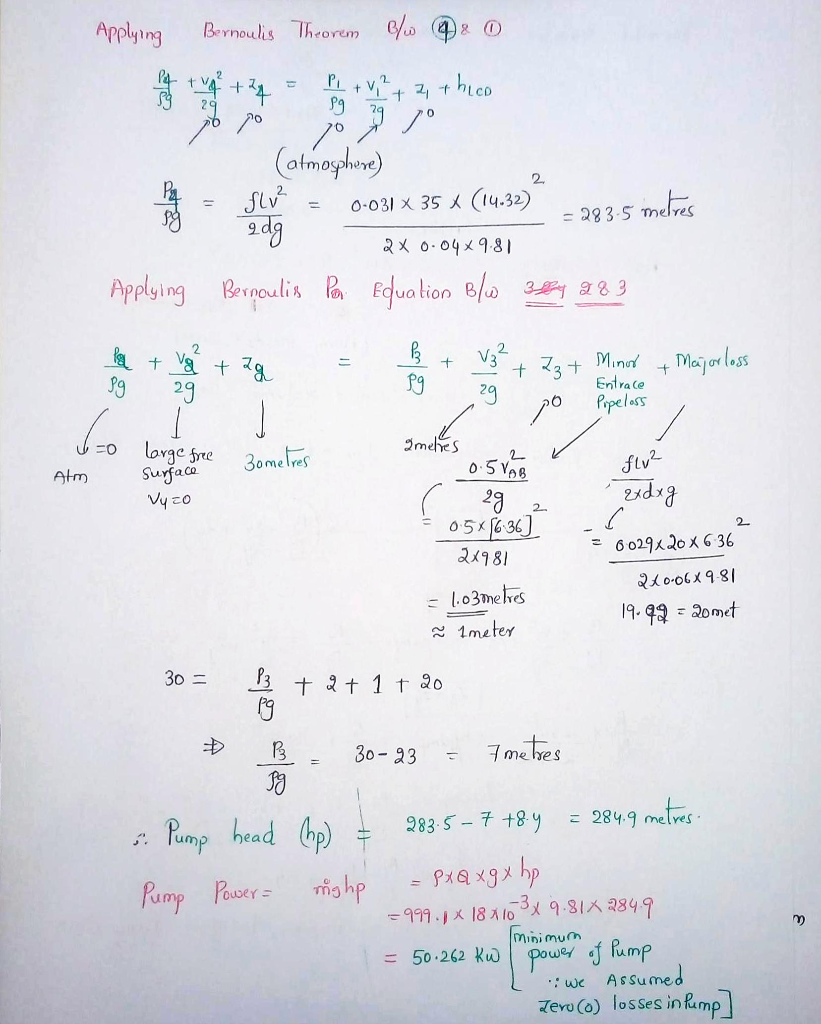#### Earn Coins

Coins can be redeemed for fabulous gifts.

Similar Homework Help Questions
• ### fluid mechanics ASAP please Problem 4 (50 points) Water is discharged from a reservoir at a rate of 18.103 m3/s using two horizontal cast iron pipes connected in series and a pump between them. T...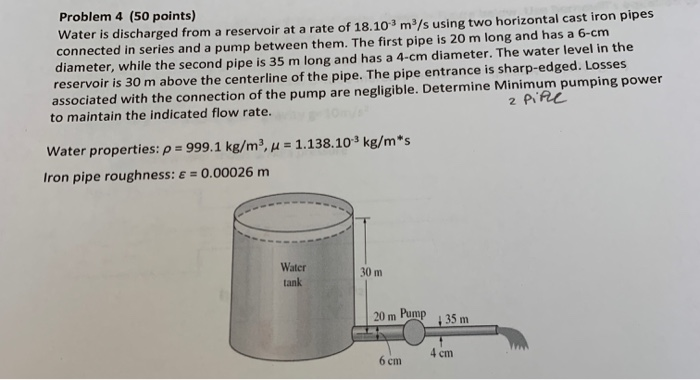fluid mechanics ASAP please Problem 4 (50 points) Water is discharged from a reservoir at a rate of 18.103 m3/s using two horizontal cast iron pipes connected in series and a pump between them. The first pipe is 20 m long and has a 6-cm diameter, while the second pipe is 35 m long and has a 4-cm diameter. The water level in the reservoir is 30 m above the centerline of the pipe. The pipe entrance is sharp-edged. Losses...

• ### Water at 15 C is drained from a large reservoir using two horizontal plastic pipes connected...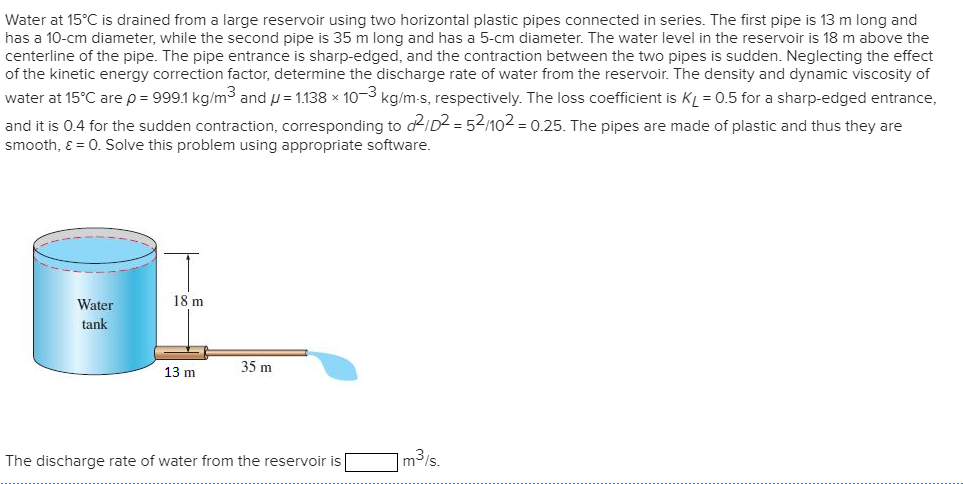Water at 15 C is drained from a large reservoir using two horizontal plastic pipes connected in series. The first pipe is 13 m long and has a 10-cm diameter, while the second pipe is 35 m long and has a 5-cm diameter. The water level in the reservoir is 18 m above the centerline of the pipe. The pipe entrance is sharp-edged, and the contraction between the two pipes is sudden. Neglecting the effect of the kinetic energy correction...

• ### Question (20 marks Analysis only required Water at 15°C is discharged freely to atmosphere from a...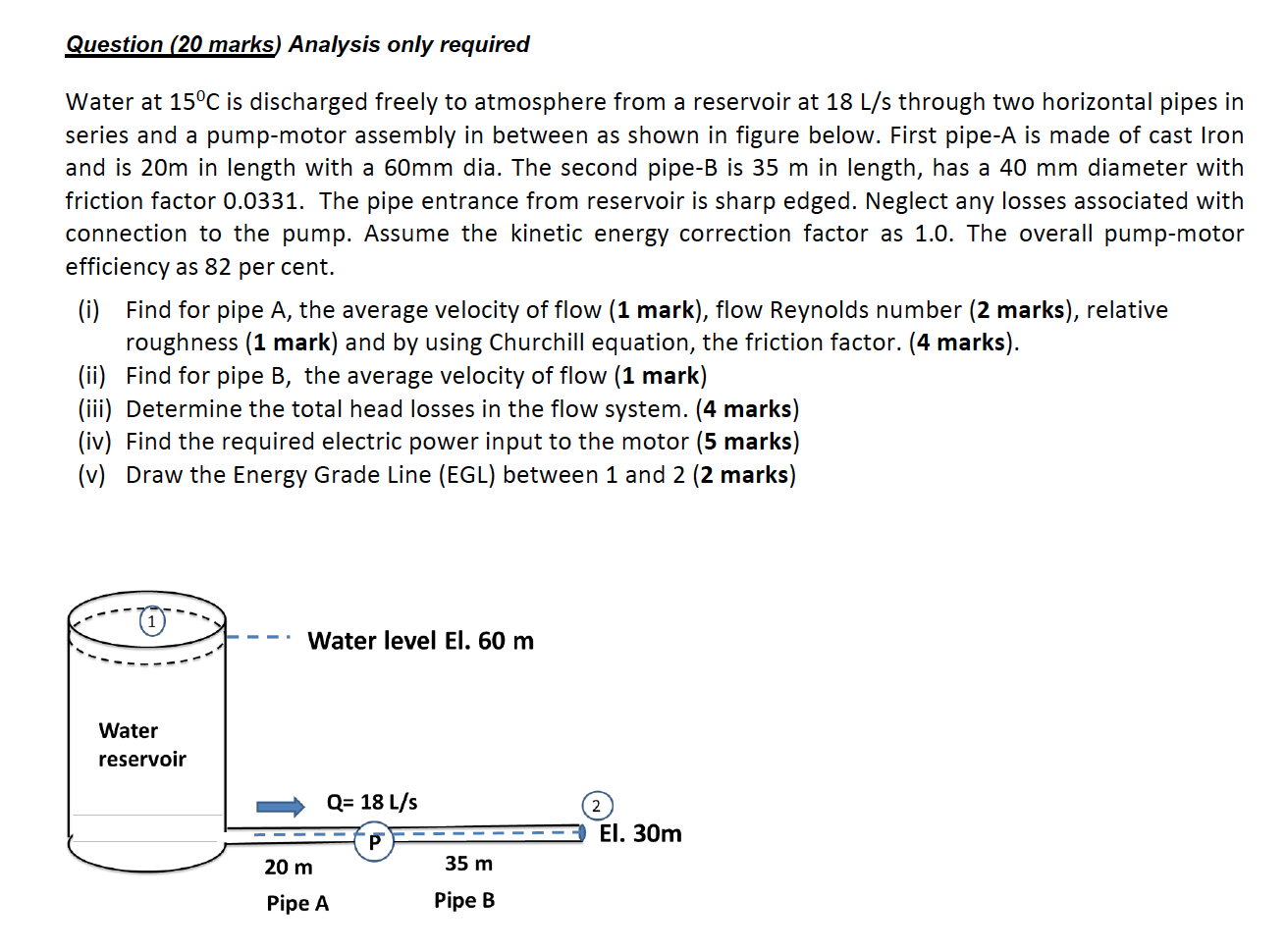Question (20 marks Analysis only required Water at 15°C is discharged freely to atmosphere from a reservoir at 18 L/s through two horizontal pipes in series and a pump-motor assembly in between as shown in figure below. First pipe-A is made of cast Iron and is 20m in length with a 60mm dia. The second pipe-B is 35 m in length, has a 40 mm diameter with friction factor 0.0331. The pipe entrance from reservoir is sharp edged. Neglect any...

• ### Paton P'S 2 107 3.6 The inlet of a cast iron pipe, 120 m long and...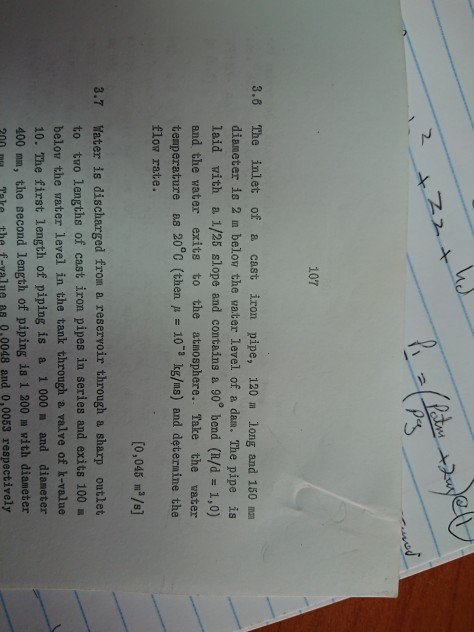Paton P'S 2 107 3.6 The inlet of a cast iron pipe, 120 m long and 150 mm a dam. The pipe is 1/25 slope and contains a 90 bend (R/d = 1,0) diameter is 2 m below the wa.ter level of laid with a and the water exits to the atmosphere. Take the water temperature B.S 20 C (then 10 kg/ms) and determine the flow rate. [0,045 m2/s] 3.7 Water is discharged from a reservoir through a sharp outlet...

• ### Water, with density of 1000 kg/m3 and kinematic viscosity of 1.0 x 10- m2/s, is pumped through a ...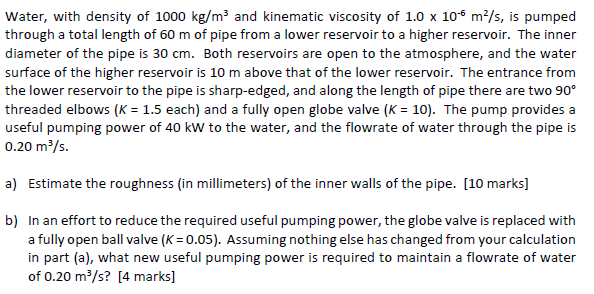Water, with density of 1000 kg/m3 and kinematic viscosity of 1.0 x 10- m2/s, is pumped through a total length of 60 m of pipe from a lower reservoir to a higher reservoir. The inner diameter of the pipe is 30 cm. Both reservoirs are open to the atmosphere, and the water surface of the higher reservoir is 10 m above that of the lower reservoir. The entrance from the lower reservoir to the pipe is sharp-edged, and along the...

• ### Two water reservoirs A and B are connected to each other through a 35m-long, 2-cm-diameter cast...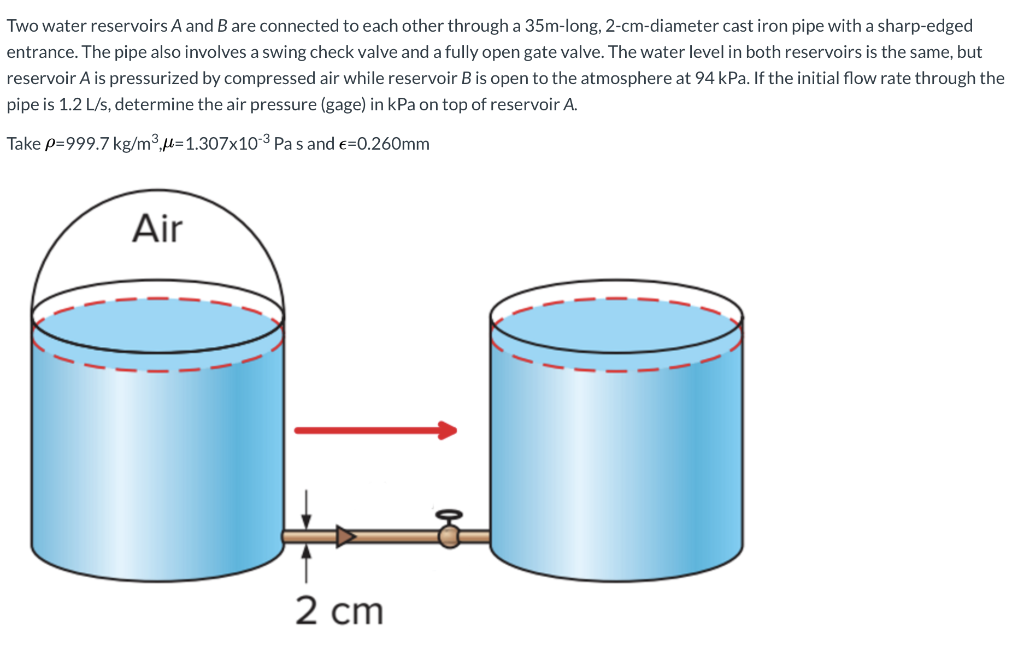Two water reservoirs A and B are connected to each other through a 35m-long, 2-cm-diameter cast iron pipe with a sharp-edged entrance. The pipe also involves a swing check valve and a fully open gate valve. The water level in both reservoirs is the same, but reservoir A is pressurized by compressed air while reservoir B is open to the atmosphere at 94 kPa. If the initial flow rate through the pipe is 1.2 L/s, determine the air pressure (gage)...

• ### Water at 15°C (ρ  = 999.1 kg/m3 and μ = 1.138 × 10−3 kg/m·s) is flowing steadily in a 34-m-long and 6-cm-diameter horizontal pipe made of stainless steel at a rate of 10 L/s. Determine the pressure d...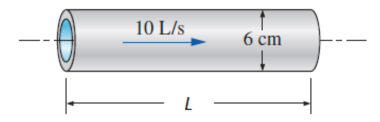Water at 15°C (ρ  = 999.1 kg/m3and μ = 1.138 × 10−3 kg/m·s) is flowing steadily in a 34-m-long and 6-cm-diameter horizontal pipe made of stainless steel at a rate of 10 L/s. Determine the pressure drop, the head loss, and the pumping power requirement to overcome this pressure drop. The roughness of stainless steel is 0.002 mm.Determine the following:A)The pressure drop in _______  kPa.B)The head loss in _______  m.C)The pumping power requirement in _______  kW.

• ### Two water reservoirs A and B are connected to each other through a 35m-long, 2-cm-diameter cast...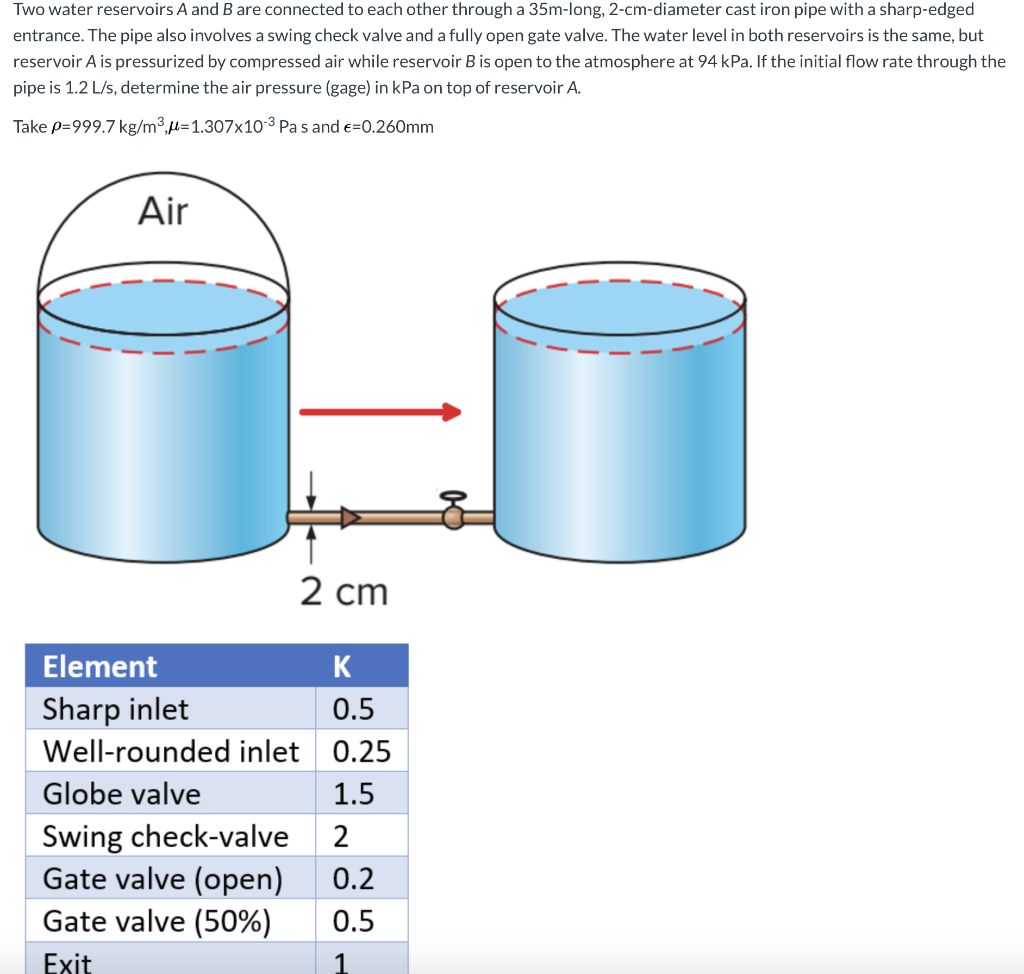Two water reservoirs A and B are connected to each other through a 35m-long, 2-cm-diameter cast iron pipe with a sharp-edged entrance. The pipe also involves a swing check valve and a fully open gate valve. The water level in both reservoirs is the same, but reservoir A is pressurized by compressed air while reservoir B is open to the atmosphere at 94 kPa. If the initial flow rate through the pipe is 1.2 L/s, determine the air pressure (gage)...

• ### Water (density = 1000 kg/m3, viscosity = 1.15 x 10-3 N-s/m2) is delivered from a large reservoir ...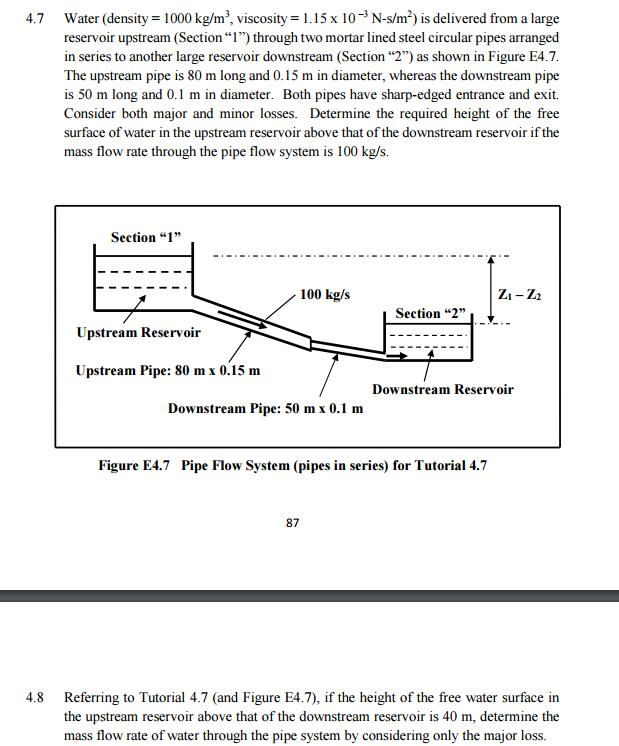Water (density = 1000 kg/m3, viscosity = 1.15 x 10-3 N-s/m2) is delivered from a large reservoir upstream (Section"1") through two mortar lined steel circular pipes arranged in series to another large reservoir downstream (Section "2") as shown in Figure E4.7 The upstream pipe is 80 m long and 0.15 m in diameter, whereas the downstream pipe is 50 m long and 0.1 m in diameter. Both pipes have sharp-edged entrance and exit. Consider both major and minor losses. Determine...

• ### 5 points Water flows from left reservoir to right reservoir in a 5 cm diameter pipe...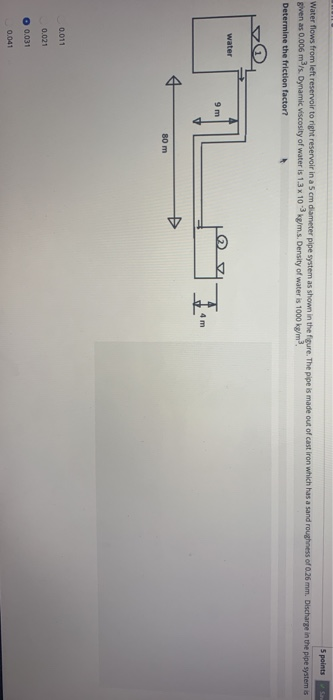5 points Water flows from left reservoir to right reservoir in a 5 cm diameter pipe system as shown in the figure. The pipe is made out of cast iron which has a sand roughness of 0.26 mm. Discharge in the pipe system is given as 0.006 m/s. Dynamic viscosity of water is 1.3 x 103 kg/m.s. Density of water is 1000 kg/m Determine the friction factor? water 9 m 4 m 80 m 0.011 0.021 O 0.031 0.041